[Next] [Up] [Previous] [Contents]
Next: Running Prolog Up: Tutorial Previous: Definitions   Contents

# Functions

Now let's take on a harder problem: defining capture-avoiding substitution. We'll define substitution as a function since we usually want to run it forward'' (i.e., given a term and a substitution, construct the result) rather than reverse''.

To declare a function write (in tutorial.apl):

func subst(tm,[(id,tm)]) = tm.

This expresses that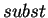is a function symbol taking two arguments: a term and a list of identifier-term pairs (i.e., the subsitution), and producing a term. Now let's define cases. Clauses for function definitions are of the form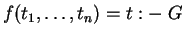, where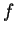is the function being defined, the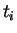are the inputs for which the clause defines a value,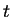is the value, and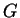is a subgoal that must be solved for the clause to apply. For example
subst(var(A),S)     = T                           :- mem((A,T),S).
subst(var(A),S)     = var A                       :- not(mem(A,_),S).
subst(app(T1,T2),S) = app(subst(T1,S),subst(T2,S)).
subst(lam(x\T),S)   = lam(x\subst(T,S))           :- x # S.

The first clause asserts that if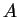is bound to a term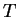in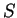thenis the result. The second, that ifis not so bound, then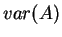is the result. The third clause just propagatespast an application. The final clause propagatespast a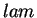, provided the bound variable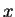is not mentioned in. Sinceappears only in an abstraction, it is always possible to satisfy this constraint by renaming(although at times renaming may not be necessary). In fact, this is what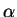Prolog does by default.

Is this definition really correct? Let's try some examples.

?- X = subst(var(a), [(a,var(b))]).
Yes.
X = var b
?- X = subst(lam(a\var(a)), [(a,var(b))]).
Yes.
X = lam (a\var(a))
?- X = subst(lam(b\var(a)), [(a,var(b))]).
Yes.
X = lam (b1\var(b))
;
No.

Note that in the final case, the bound variable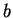has been freshened to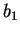. Remember that when a name is used in an abstraction, we lose control over its specific value since the abstraction really represents an equivalence class of-equivalent terms. The last example also verifies that a simple form of variable capture is actually impossible. That is, it's not the case thathappens to get the right answer the first time it succeeds; in fact, no alternative wrong answers are possible.

Now this definition ofhas some disadvantages: for example the first two clauses overlap and may repeat the membership test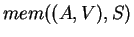. We can make this more efficient usingProlog's if-then-else'' construct:

subst(var A,S) = T :- mem ((A,V),S) -> T = V | T = var(A).

Similarly, we can collapse the other two clauses into one completely general case using disjunction:
subst(T,A) = T' :- ( T = var(A),
if mem((A,V),S)
then T = V
else T = var(A)
; T = app(T1,T2),
T' = app(subst(T1,S),subst(T2,S)))
; T = lam(x\T1),
x # S,
T' = lam(x\subst(T1,S)))


Another alternative would be to replace the call to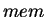with a substitution-specific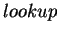function:

func lookup(id,[(id,exp)]) = exp.
lookup(A,[]) = var A.
lookup(A,[(A,V)|S]) = V.
lookup(A,[(B,V)|S]) = lookup(A,S).
...
subst(var A,S) = lookup(A,S).


Both this and the earlier definition have the potential disadvantage that if duplicate bindings are present in a substitution (e.g.,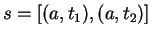), thenorcan succeed with multiple possible answers. We therefore regard substitutions to be well-formed only if there are no such duplicates.

[Next] [Up] [Previous] [Contents]
Next: Running Prolog Up: Tutorial Previous: Definitions   Contents
James Cheney 2003-10-23